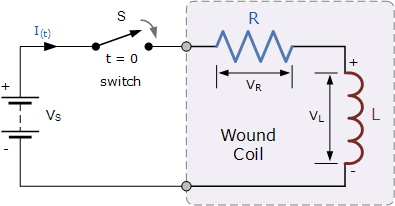# What is the current equation for an inductor and battery series circuit?5 years ago

Majority of coils that you see in the real world, whether they are solenoids, relays or any other similar component always exhibit certain amount of resistance because the copper wire has a resistive value. In such a case, the simple coil can then be considered as an Inductance in series with a resistance. This may be termed as the RL Series Circuit.
We have shown the RL Circuit in the figure given below. The circuit basically consists of an inductor of inductance L connected in series with a resistor of resistance R. This ‘R’ is the DC resistive value of the loops that is utilized in the preparation of the coil of the inductor.As it is visible form the figure above, the circuit is attached to a battery and switch. The switch ‘S’ is assumed to be open till it is closed at time t = 0. It will then remain constantly closed thus producing a "step response" type voltage input. The current ‘i’ though flows through the circuit, but will not rise to its maximum value of Imax.

This restriction is basically due to the existence of the self-induced emf inside the inductor due to the growth of magnetic flux. After some time, the influence of the self- induced emf is nullified by the voltage source. The flow of current then becomes constant and the induced current and field are reduced to zero.

5 years ago

In a simple L-R circuit,

If apply KVL we get,

L*di/dt + iR = 0
integeratig both sides via variable separable method,
we get,
di/i = -R/Ldt
integerate both sides now,

log(i) = -(R/L)t
i = e^(-R/L)*t